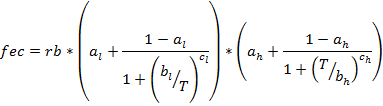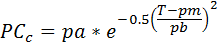SORTIE-ND
Software for spatially-explicit simulation of forest dynamics

# Stochastic Double Logistic Temp Dependent Neighborhood Disperse behavior

This behavior calculates seed density based on annual mean temperature and the basal area of neighborhood adults.

### Parameters for this behavior

Parameter nameDescription
Minimum DBH for Reproduction, in cmThe minimum DBH at which a tree can reproduce. This value does not have to match the Minimum adult DBH.
Colonization PAPA in the function determing probability of colonization.
Colonization PBPB in the function determing probability of colonization.
Colonization PMPM in the function determing probability of colonization.
Cumulative Colonization Probability Period (years)The period over which the probability of colonization is cumulative.
Fecundity Temperature Effect AlAl in the temperature effect portion of fecundity. Ignored if "Use Temperature-Dependent Fecundity?" is set to false.
Fecundity Temperature Effect BlBl in the temperature effect portion of fecundity. Ignored if "Use Temperature-Dependent Fecundity?" is set to false.
Fecundity Temperature Effect ClCl in the temperature effect portion of fecundity. Ignored if "Use Temperature-Dependent Fecundity?" is set to false.
Fecundity Temperature Effect AhAh in the temperature effect portion of fecundity. Ignored if "Use Temperature-Dependent Fecundity?" is set to false.
Fecundity Temperature Effect BhBh in the temperature effect portion of fecundity. Ignored if "Use Temperature-Dependent Fecundity?" is set to false.
Fecundity Temperature Effect ChCh in the temperature effect portion of fecundity. Ignored if "Use Temperature-Dependent Fecundity?" is set to false.
Max Search Distance for Conspecific Adults (m)The maximum distance to search for conspecific adults when determining number of seeds.
Original Analysis Plot Size (m2)The size of the seedling plots in the original parameter analysis, in square meters. This is used to scale the per-grid-cell seed values.
Presence Threshold in Absence of Adults (0-1)Threshold value, between 0 and 1, of the presence test function above which a species will be allowed to disperse in the absence of parents. 0 always includes a species, 1 always excludes it.
Seeds RARA in the final seed calculation.
Seeds RBRB in the final seed calculation.
Use Temperature-Dependent Fecundity?Whether or not to use the temperature effect portion of fecundity. If this is false, fecundity = RB.

### How it works

The mean number of seeds in each cell of the Dispersed Seeds grid is calculated as:

Seeds = RA + fec * BAC

where:

• Seeds is the mean number of seeds in the cell
• RA is the Seeds RA parameter
• BAC is the total basal area of neighborhood conspecific adults in square meters within a radius of Max Search Distance for Conspecific Adults (m)
• fec is a fecundity term (more below)

The mean seed value, above, is used as the mean on a Poisson random draw. The final seed number assigned to the grid is:

Final seeds = seeds * (CA/OA) * Y

where:

• seeds is the result of the Poisson draw
• CA is the area of the grid cell, in square meters
• OA is the Original Analysis Plot Size (m2) parameter
• Y is the number of years per timestep

Note that this behavior works on a per-grid-cell basis, rather than calculating seed densities on a per-square-meter basis. This is because the Poisson function is influenced by seed grid cell size. You can use the Original Analysis Plot Size (m2) parameter to scale results to original analysis plot size, but for best results, the seed grid cell size should be set to the same or slightly larger than the original analysis seed plot size.

"fec" is a per capita seedling production (fecundity) term. It is a function of temperature and is calculated as:where:

• RB is the Seeds RB parameter
• T is the annual mean temperature, in degrees Kelvin, calculated from the value in degrees C in the Plot
• al is the Fecundity Temperature Effect Al parameter
• bl is the Fecundity Temperature Effect Bl parameter
• cl is the Fecundity Temperature Effect Cl parameter
• ah is the Fecundity Temperature Effect Ah parameter
• bh is the Fecundity Temperature Effect Bh parameter
• ch is the Fecundity Temperature Effect Ch parameter

The temperature-dependent portion of fecundity is optional. If the parameter Use Temperature-Dependent Fecundity? is set to false, then fec = RB.

### Colonization

Note that the RA parameter in the mean seeds equation is an intercept, potentially allowing bath rain of seeds for species for which there are no parents present. To manage this, the behavior uses a colonization test. The cumulative probability of colonization is:where:

• PCc is the cumulative probability of colonization
• pa is the Colonization PA parameter
• pb is the Colonization PB parameter
• pm is the Colonization PM parameter
• T is the annual mean temperature, in degrees Celsius, as entered for the Plot

PCc is the cumulative probability of colonization over an arbitrary time period T. PCc is used to calculate the annualized probability of colonization as:

PCa = 1 - [(1 - PCc)(1/t)]

where:

• PCa is the annualized probability of colonization
• T is the Cumulative Colonization Probability Period (years) parameter

The behavior compares PCa to a random number to determine whether this species is allowed to colonize this timestep. If so, it disperses as described above.

### How to apply it

Apply this behavior to adults of the species you wish to use.

-->